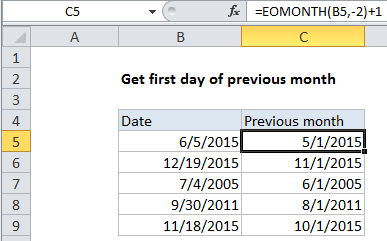## Excel Office

Excel How Tos, Tutorials, Tips & Tricks, Shortcuts

# Get first day of previous month in Excel

To get the first day of the previous month for a given date, you can use a simple formula based on the EOMONTH function.

## Formula

`=EOMONTH(date,-2)+1`## Explanation

In the example shown, the formula in cell B5 is:

`=EOMONTH(B5,-2)+1`

In the example shown, months is supplied as -2, which causes EOMONTH to return 4/30/2015. Then, 1 day is added to get 5/1/2015.

Worked Example:   How to calculate working days left in month in Excel

The EOMONTH function returns the last day of a month based on a given date. The 2nd argument is months, which specifies how many months in the future or past to move before returning the last day. By traveling back 2 months, then adding one day, we can calculate the first day of the previous month from any given date.

Worked Example:   Get last weekday in month in Excel

Worked Example:   Steps to create Dynamic calendar grid in Excel